# text

Add text descriptions to data points

## Syntax

text(x,y,txt)
text(x,y,z,txt)
text(___,Name,Value)
text(ax,___)
t = text(___)

## Description

example

text(x,y,txt) adds a text description to one or more data points in the current axes using the text specified by txt. To add text to one point, specify x and y as scalars. To add text to multiple points, specify x and y as vectors with equal length.
text(x,y,z,txt) positions the text in 3-D coordinates.

example

text(___,Name,Value) specifies Text object properties using one or more name-value pairs. For example, 'FontSize',14 sets the font size to 14 points. You can specify text properties with any of the input argument combinations in the previous syntaxes. If you specify the Position and String properties as name-value pairs, then you do not need to specify the x, y, z, and txt inputs.
text(ax,___) creates the text in the Cartesian, polar, or geographic axes specified by ax instead of in the current axes (gca). The option ax can precede any of the input argument combinations in the previous syntaxes.

example

t = text(___) returns one or more text objects. Use t to modify properties of the text objects after they are created. For a list of properties and descriptions, see Text Properties. You can specify an output with any of the previous syntaxes.

## Examples

collapse all

Plot a sine curve. At the point $\left(\pi ,0\right)$, add the text description $\mathrm{sin}\left(\pi \right)$. Use the TeX markup \pi for the Greek letter $\pi$. Use \leftarrow to display a left-pointing arrow.

x = 0:pi/20:2*pi; y = sin(x); plot(x,y) text(pi,0,'\leftarrow sin(\pi)')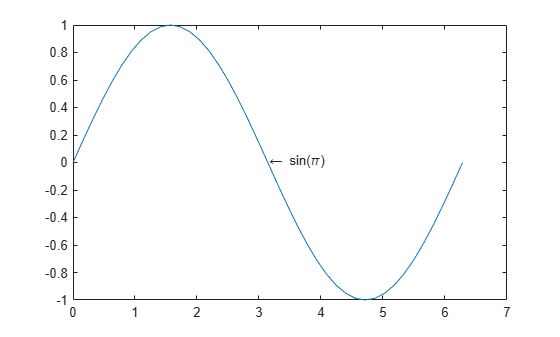For a list of Greek characters and other TeX markup, see the Interpreter property description.

Plot a line. Add the same text to two points along the line.

x = linspace(-5,5); y = x.^3-12*x; plot(x,y) xt = [-2 2]; yt = [16 -16]; str = 'dy/dx = 0'; text(xt,yt,str)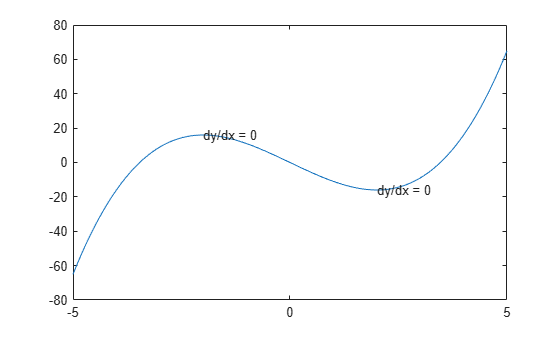Add different text to each point by specifying str as a cell array.

x = linspace(-5,5); y = x.^3-12*x; plot(x,y) xt = [-2 2]; yt = [16 -16]; str = {'local max','local min'}; text(xt,yt,str)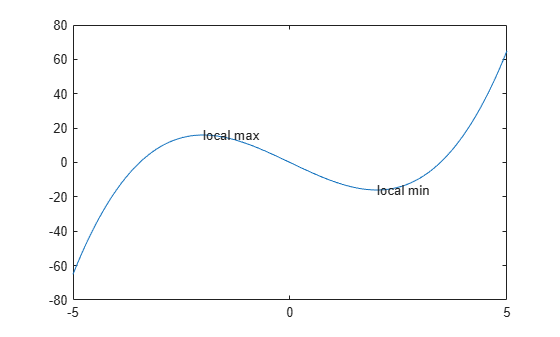Create a line plot and add one text description to the axes. Display multiline text by specifying str as a cell array.

plot(1:10) str = {'A simple plot','from 1 to 10'}; text(2,7,str)Create a line plot and add two text descriptions to the axes. When adding multiple text descriptions to the axes, display multiline text by specifying nested cell arrays.

plot(1:10) str = {{'A simple plot','from 1 to 10'},'y = x'}; text([2 8],[7 7],str)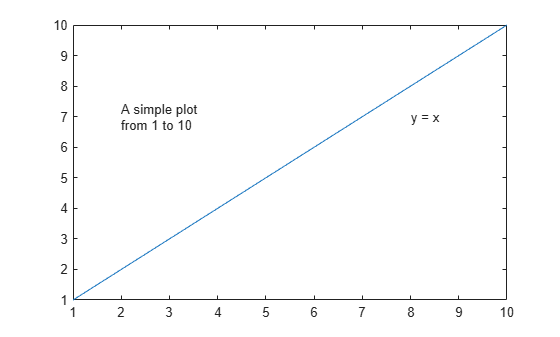Create a line plot and add a text description to the axes. Use red, size 14 font.

plot(1:10) text(2,8,'A Simple Plot','Color','red','FontSize',14)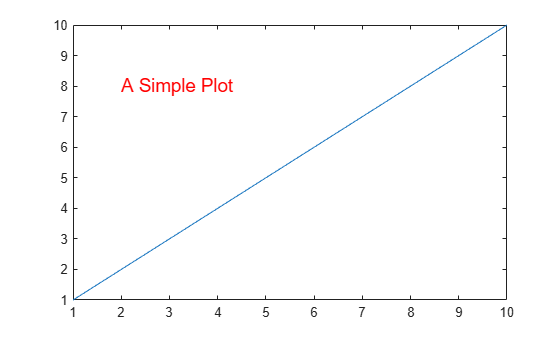Create a line plot and add two text descriptions along the line. Return the text objects, t.

x = linspace(-5,5); y = x.^3-12*x; plot(x,y) t = text([-2 2],[16 -16],'dy/dx = 0')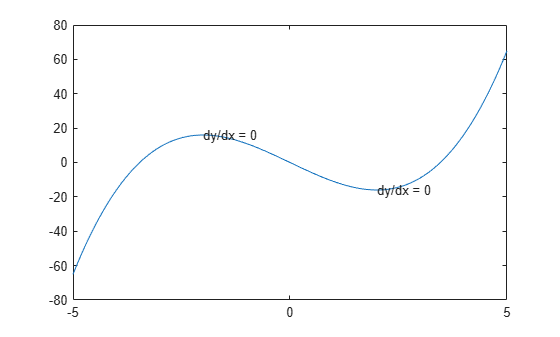t = 2x1 Text array: Text (dy/dx = 0) Text (dy/dx = 0) 

The text function creates one text object for each text description. Thus, t contains two text objects.

Change the color and font size for the first text object using t(1). Use dot notation to set properties. If you are using an earlier release, use the set function instead.

t(1).Color = 'red'; t(1).FontSize = 14;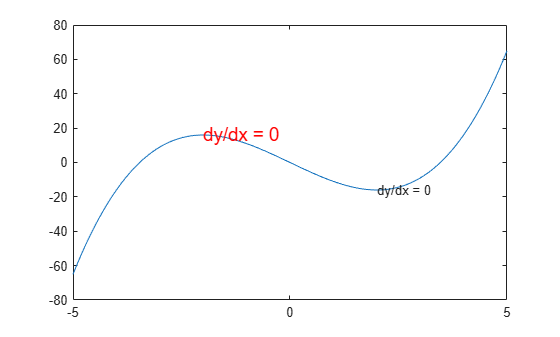## Input Arguments

collapse all

First coordinate, specified in one of these forms:

• Scalar — Add text to a single point.

• Vector — Add text to multiple points.

The interpretation of the first coordinate depends on the type of axes:

• For Cartesian axes, the first coordinate is x-axis position in data units. To change units, set the Units property for the Text object.

• For polar axes, the first coordinate is the polar angle θ in radians.

• For geographic axes, the first coordinate is latitude in degrees.

x and y must be equal sizes.

To control the placement of the text with relation to the specified point, use the HorizontalAlignment, VerticalAlignment, and Extent properties of the Text object.

Data Types: single | double | int8 | int16 | int32 | int64 | uint8 | uint16 | uint32 | uint64 | categorical | datetime | duration

Second coordinate, specified in one of these forms:

• Scalar — Add text to a single point.

• Vector — Add text to multiple points.

The interpretation of the second coordinate depends on the type of axes:

• For Cartesian axes, the second coordinate is y-axis position in data units. To change units, set the Units property for the Text object.

• For polar axes, the second coordinate is the radius in data units.

• For geographic axes, the second coordinate is longitude in degrees.

x and y must be equal sizes.

To control the placement of the text with relation to the specified point, use the HorizontalAlignment, VerticalAlignment, and Extent properties of the Text object.

Data Types: single | double | int8 | int16 | int32 | int64 | uint8 | uint16 | uint32 | uint64 | categorical | datetime | duration

Third coordinate, specified in one of these forms:

• Scalar — Add text to a single point.

• Vector — Add text to multiple points.

The interpretation of the third coordinate depends on the type of axes:

• For Cartesian axes, the third coordinate is z-axis position in data units. To change units, set the Units property for the Text object.

• For polar and geographic axes, the third coordinate affects the layering of text on the axes.

x, y, and z must be equal sizes.

To control the placement of the text with relation to the specified point, use the HorizontalAlignment, VerticalAlignment, and Extent properties of the Text object.

Data Types: single | double | int8 | int16 | int32 | int64 | uint8 | uint16 | uint32 | uint64 | categorical | datetime | duration

Text to display, specified as a character vector, string scalar, character array, string array, cell array, or categorical array.

#### Text for Single Data Point

To add text to a single point, specify txt as a character vector or string scalar. For example, text(.5,.5,'my text').

For multiline text:

• Use a cell array, where each cell contains a line of text. For example, text(.5,.5,{'first','second'}).

• Use a string array, where each element is a line of text. For example, text(.5,.5,["first","second"]).

• Use a character array with multiple rows, where each row contains the same number of characters. For example, text(.5,.5,['first '; 'second']).

• Use sprintf to create a new line character. For example, text(.5,.5,sprintf('first \nsecond')).

If you specify the text as a categorical array, MATLAB® uses the values in the array, not the categories.

#### Text for Multiple Data Points

To display the same text at each location, specify txt as a character vector or string. For example, text([0 1],[0 1],'my text').

To display different text at each location, use a cell array. For example, text([0 1],[0 1],{'first','second'}).

For multiline text, use nested cell arrays. For example, text([0 1],[0 1],{{'first','new line'},'second'}).

#### Greek Letters, Special Characters, and Numeric Variables

To include special characters, such as superscripts, subscripts, Greek letters, or mathematical symbols, use TeX markup. For a list of supported markup, see the Interpreter property.

To include numeric variables in the text, use the num2str function.

v = 42; txt = ['The value is ',num2str(v)];

Target axes, specified as an Axes object, a PolarAxes object, or a GeographicAxes object. If you do not specify the axes, then text uses the current axes.

### Name-Value Pair Arguments

Specify optional comma-separated pairs of Name,Value arguments. Name is the argument name and Value is the corresponding value. Name must appear inside quotes. You can specify several name and value pair arguments in any order as Name1,Value1,...,NameN,ValueN.

Example: text(.5,.5,'my text','FontSize',14,'Color','red')

Note

The properties listed here are only a subset. For a full list, see Text Properties.

Font size, specified as a scalar value greater than zero in point units. The default font size depends on the specific operating system and locale. One point equals 1/72 inch. To change the font units, use the FontUnits property.

Example: 12

Data Types: single | double | int8 | int16 | int32 | int64 | uint8 | uint16 | uint32 | uint64

Character thickness, specified as 'normal' or 'bold'.

MATLAB uses the FontWeight property to select a font from those available on your system. Not all fonts have a bold weight. Therefore, specifying a bold font weight can still result in the normal font weight.

Font name, specified as a supported font name or 'FixedWidth'. To display and print text properly, you must choose a font that your system supports. The default font depends on your operating system and locale.

To use a fixed-width font that looks good in any locale, use 'FixedWidth'. The fixed-width font relies on the root FixedWidthFontName property. Setting the root FixedWidthFontName property causes an immediate update of the display to use the new font.

Text color, specified as an RGB triplet, a hexadecimal color code, a color name, or a short name. The default value of [0 0 0] corresponds to black.

For a custom color, specify an RGB triplet or a hexadecimal color code.

• An RGB triplet is a three-element row vector whose elements specify the intensities of the red, green, and blue components of the color. The intensities must be in the range [0,1]; for example, [0.4 0.6 0.7].

• A hexadecimal color code is a character vector or a string scalar that starts with a hash symbol (#) followed by three or six hexadecimal digits, which can range from 0 to F. The values are not case sensitive. Thus, the color codes '#FF8800', '#ff8800', '#F80', and '#f80' are equivalent.

Alternatively, you can specify some common colors by name. This table lists the named color options, the equivalent RGB triplets, and hexadecimal color codes.

Color NameShort NameRGB TripletHexadecimal Color CodeAppearance
'red''r'[1 0 0]'#FF0000''green''g'[0 1 0]'#00FF00''blue''b'[0 0 1]'#0000FF''cyan' 'c'[0 1 1]'#00FFFF''magenta''m'[1 0 1]'#FF00FF''yellow''y'[1 1 0]'#FFFF00''black''k'[0 0 0]'#000000''white''w'[1 1 1]'#FFFFFF''none'Not applicableNot applicableNot applicableNo color

Here are the RGB triplets and hexadecimal color codes for the default colors MATLAB uses in many types of plots.

[0 0.4470 0.7410]'#0072BD'[0.8500 0.3250 0.0980]'#D95319'[0.9290 0.6940 0.1250]'#EDB120'[0.4940 0.1840 0.5560]'#7E2F8E'[0.4660 0.6740 0.1880]'#77AC30'[0.3010 0.7450 0.9330]'#4DBEEE'[0.6350 0.0780 0.1840]'#A2142F'Example: 'blue'

Example: [0 0 1]

Example: '#0000FF'

Horizontal alignment of the text with respect to the x value in the Position property, specified as one of the values in this table. The vertical line indicates where the x value lies in relation to the text.

ValueResult
'left' (default)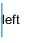'center'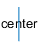'right'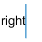Location of the text, specified as a two-element vector of the form [x y] or a three-element vector of the form [x y z]. If you omit the third element, z, then MATLAB sets it to 0.

Specify the position using numeric values. To convert datetime or duration values to the appropriate numeric values for a particular coordinate direction, see ruler2num.

By default, the position value is defined in data units. To change the units, use the Units property.

Example: [0.5 0.5 0]

Data Types: double | single | int8 | int16 | int32 | int64 | uint8 | uint16 | uint32 | uint64

Position units, specified as one of the values in this table.

UnitsDescription
'data' (default)Data coordinates.
'normalized' Normalized with respect to the axes. The lower left corner of the axes maps to (0,0) and the upper right corner maps to (1,1).
'inches'Inches.
'centimeters'Centimeters.
'characters'

Based on the default system font character size.

• Character width = width of letter x.

• Character height = distance between the baselines of two lines of text.

'points'Points. One point equals 1/72 inch.
'pixels'

Pixels.

Starting in R2015b, distances in pixels are independent of your system resolution on Windows® and Macintosh systems:

• On Windows systems, a pixel is 1/96th of an inch.

• On Macintosh systems, a pixel is 1/72nd of an inch.

On Linux® systems, the size of a pixel is determined by your system resolution.

All units, except for 'data', are measured from the lower left corner of the axes. This property affects the Position and Extent properties.

If you specify the Position and Units properties as Name,Value pairs when creating the Text object, then the order of specification matters. To define the position with particular units, set the Units property before the Position property.

Text interpreter, specified as one of these values:

• 'tex' — Interpret characters using a subset of TeX markup.

• 'latex' — Interpret characters using LaTeX markup.

• 'none' — Display literal characters.

#### TeX Markup

By default, MATLAB supports a subset of TeX markup. Use TeX markup to add superscripts and subscripts, modify the font type and color, and include special characters in the text.

Modifiers remain in effect until the end of the text. Superscripts and subscripts are an exception because they modify only the next character or the characters within the curly braces. When you set the interpreter to 'tex', the supported modifiers are as follows.

ModifierDescriptionExample
^{ }Superscript'text^{superscript}'
_{ }Subscript'text_{subscript}'
\bfBold font'\bf text'
\itItalic font'\it text'
\slOblique font (usually the same as italic font)'\sl text'
\rmNormal font'\rm text'
\fontname{specifier}Font name — Replace specifier with the name of a font family. You can use this in combination with other modifiers.'\fontname{Courier} text'
\fontsize{specifier}Font size —Replace specifier with a numeric scalar value in point units.'\fontsize{15} text'
\color{specifier}Font color — Replace specifier with one of these colors: red, green, yellow, magenta, blue, black, white, gray, darkGreen, orange, or lightBlue.'\color{magenta} text'
\color[rgb]{specifier}Custom font color — Replace specifier with a three-element RGB triplet.'\color[rgb]{0,0.5,0.5} text'

This table lists the supported special characters for the 'tex' interpreter.

Character SequenceSymbolCharacter SequenceSymbolCharacter SequenceSymbol

\alpha

α

\upsilon

υ

\sim

~

\angle

\phi\leq

\ast

*

\chi

χ

\infty

\beta

β

\psi

ψ

\clubsuit

\gamma

γ

\omega

ω

\diamondsuit

\delta

δ

\Gamma

Γ

\heartsuit

\epsilon

ϵ

\Delta

Δ

\spadesuit

\zeta

ζ

\Theta

Θ

\leftrightarrow

\eta

η

\Lambda

Λ

\leftarrow

\theta

θ

\Xi

Ξ

\Leftarrow

\vartheta

ϑ

\Pi

Π

\uparrow

\iota

ι

\Sigma

Σ

\rightarrow

\kappa

κ

\Upsilon

ϒ

\Rightarrow

\lambda

λ

\Phi

Φ

\downarrow

\mu

µ

\Psi

Ψ

\circ

º

\nu

ν

\Omega

Ω

\pm

±

\xi

ξ

\forall

\geq

\pi

π

\exists

\propto

\rho

ρ

\ni

\partial

\sigma

σ

\cong

\bullet

\varsigma

ς

\approx

\div

÷

\tau

τ

\Re

\neq

\equiv

\oplus

\aleph

\Im

\cup

\wp

\otimes

\subseteq

\oslash

\cap

\in

\supseteq

\supset

\lceil

\subset

\int

\cdot

·

\o

ο

\rfloor

\neg

¬

\nabla

\lfloor

\times

x

\ldots

...

\perp

\surd

\prime

´

\wedge

\varpi

ϖ

\0

\rceil

\rangle

\mid

|

\vee

\langle

\copyright

#### LaTeX Markup

To use LaTeX markup, set the interpreter to 'latex'. For inline mode, surround the markup with single dollar signs ($). For display mode, surround the markup with double dollar signs ($$). LaTeX ModeExampleResult Inline '\int_1^{20} x^2 dx'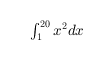Display '$$\int_1^{20} x^2 dx$\$'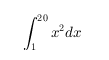The displayed text uses the default LaTeX font style. The FontName, FontWeight, and FontAngle properties do not have an effect. To change the font style, use LaTeX markup.

The maximum size of the text that you can use with the LaTeX interpreter is 1200 characters. For multiline text, this reduces by about 10 characters per line.

For examples that use TeX and LaTeX, see Greek Letters and Special Characters in Chart Text. For more information about the LaTeX system, see The LaTeX Project website at https://www.latex-project.org/.

## Output Arguments

collapse all

Text objects. Use the elements of t to modify a specific Text object after it is created. For a list of properties, see Text Properties.

## Tips

• By default, the Clipping property for text objects is set to 'off' so the text might appear outside the axes. To clip the text to the axes boundaries, set the property to 'on'.

### Topics

Introduced before R2006a